【一猫汽车网】在汽车工业史上，德国宝沃汽车占据着举足轻重的地位。在其巅峰时期甚至成为了德国创新的象征。

【一猫汽车网】在汽车工业史上，德国宝沃汽车占据着举足轻重的地位。在其巅峰时期甚至成为了德国创新的象征。它的缔造者Carl F.W.Borgward（卡尔·宝沃）是世界公认的一位有天赋的设计师、一位有创造力的工程师、一位嗅觉敏锐的企业家。但事实上，他更像是一位随性的狂人，在许多不经意的偶然中，抑或是云淡风轻的谈笑间，做出看似难以想象的决定，却又凭借超强的行动力硬是让梦想照进现实。凭着对汽车的热爱、偏执和无所畏惧的大胆尝试，缔造了富有创新精神的汽车帝国，以60%的出口份额成为德国第三大汽车生产制造商，销量率先突破百万，成就了20世纪汽车工业传奇。

很难找到一张照片里的Carl F.W.Borgward（卡尔·宝沃）没有手持一根点燃的雪茄。作为德国经济奇迹的化身，雪茄逐渐成为了卡尔·宝沃的一个符号。甚至形成了他的标志动作：右手持雪茄，左臂下夹着雪茄烟袋，这在某种程度上限制了他双手的活动幅度，以至他在问候别人时不是伸出手掌而是叉开的手指，不少人甚至指责其傲慢无礼。在他60 岁的生日宴会上，一位记者获悉了他和雪茄的缘分：“在15岁时，住在隔壁的一位军需官每个月都会给我带一支纯正的哈瓦那雪茄。从此雪茄就成为了我生活的一部分，它带给我灵感。”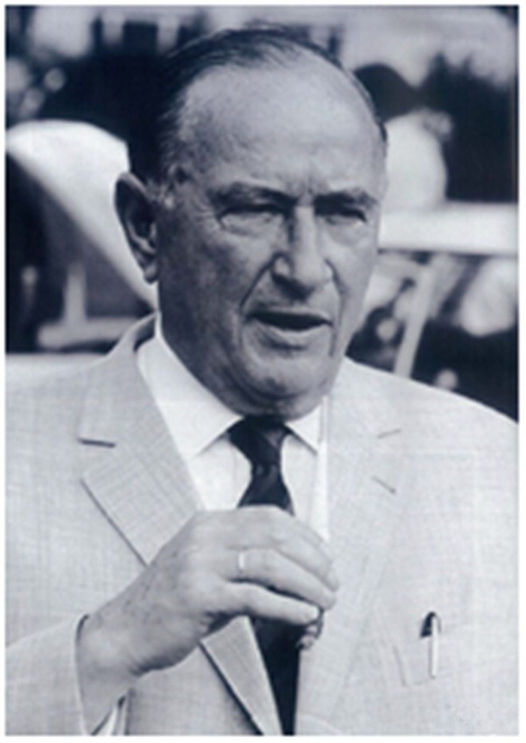回顾BORGWARD（宝沃）汽车的发展史，之所以能取得令人瞩目的成就，离不开卡尔·宝沃强大灵感的加持。在工作中，卡尔·宝沃最喜欢与工程师深入交流，并将其创新的想法转化成最佳的解决方案，尤其在车身设计方面，卡尔·宝沃更有着独特的美学视角。1953年的一天，宝沃汽车决定生产Hansa1500和1800的继任车型，但新车的设计却遭遇瓶颈。“那天，他默默地注视着样车，一根接一根地抽着雪茄，为达到镀铬抛光的最佳效果，他就这样思考了一整天，然后Isabella诞生了，它拥有超越想象的美。”BORGWARD（宝沃）汽车的工程师们如是说。不知雪茄是否真的为卡尔·宝沃天马行空的幻想和突破创新的能力助力颇多，但卡尔·宝沃手持雪茄寻找设计灵感的剪影定格在了汽车工业的发展史中。1949年，卡尔·宝沃大胆地引入美国“浮筒”设计风格打造全新Hansa1500，一举开启了德国汽车的“文艺复兴”时代；1954年，卡尔·宝沃最伟大的作品Isabella（伊莎贝拉）诞生。全新的发动机、底盘和车身让BORGWARD（宝沃）脱胎换骨，而这次突破正是受到了卡尔·宝沃强烈个人审美情趣的影响。1956年，Isabella COUPE双门轿跑更被冠以“经济奇迹时期的德国梦想之车”的称号，完全俘获了欧洲汽车消费者的芳心。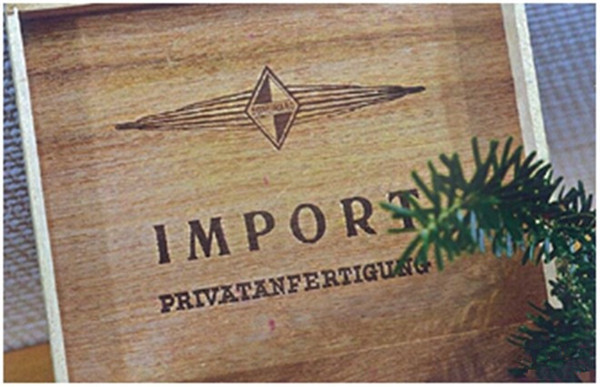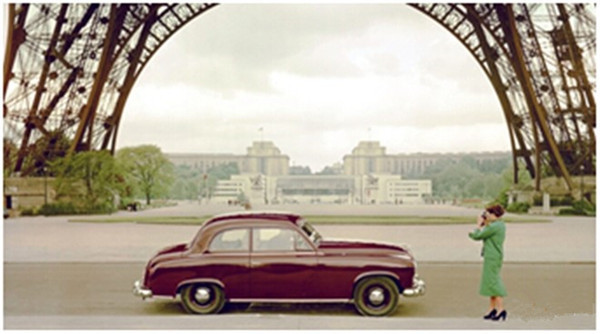Hansa 1500

不得不说，如果在卡尔·宝沃15岁时，没有一位军需官把雪茄介绍给他，汽车世界史上是否会出现这样一位奇才，难以预料。尽管中性化的趣味正在成为时尚主流，然而在男人们心中，总是多少保留着一点来自基因的英雄主义。卡斯特罗、丘吉尔、海明威、卡尔·宝沃用近乎一辈子的时间和雪茄相伴相随，在让自己成为一个个文化符号、政治符号、国家符号的同时，也让雪茄成为他们作为这个符号不可分割的一部分——成熟、冷静、坚韧且充满灵感。

BORGWARD（宝沃）公司历史中最具特色的当数直升机项目，而这个项目的启动离不开卡尔·宝沃的前瞻和突破创新的精神。作为帮助人类延伸脚步的工具发明者，卡尔·宝沃早早便将视线投向天空。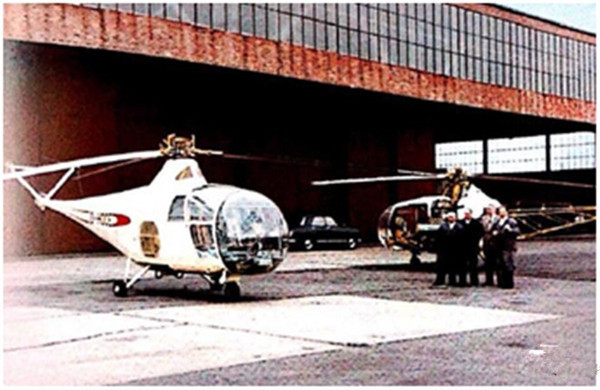卡尔·宝沃在一次朋友聚会中表示，直升机将长期代替运输行业中的汽车。此言一出，朋友们哄堂大笑，在20世纪50年代前，直升机刚刚具备试飞的能力，进行大规模运输简直是天方夜谭。但事实上，卡尔·宝沃的想法并非空穴来风，他前瞻性地察觉到当时德国较差的道路状况以及陆路交通相对较低的效率。众人的哄笑反而刺激原本处于观望的卡尔·宝沃坚定了制造宝沃直升机的信念，于是BORGWARD（宝沃）公司历史中最具特色的直升机项目当即启动。媒体采访他：为什么要制造直升飞机？他轻描淡写地说：“因为这让我觉得有趣。”而事实上，这个选择自然离不开执著的梦想和倔强的自信。在1956年至1961年间，在卡尔·宝沃和著名飞机设计师Henrich Focke的带领下，BORGWARD制造的直升机几乎实现了量产。将农林业部门、公安、海关、邮政和救护等部门统统纳入他的直升机潜在客户群。

一个女人成就一段传奇

20世纪50年代，德国中产阶级开始出现，在浪漫主义的影响下，他们开始了对“新生活、新价值”的思考。作为当时德国三大汽车生产制造商之一，BORGWARD（宝沃）汽车决定推出一款拥有全新设计思路的车型，以迎合新兴中产阶级以及年轻人的审美意趣。1954年，BORGWARD（宝沃）汽车最伟大的作品Isabella应时而生。这个作品从设计和技术角度都无懈可击，而它的命名更为其锦上添花，在汽车历史中书写了浓重的一笔。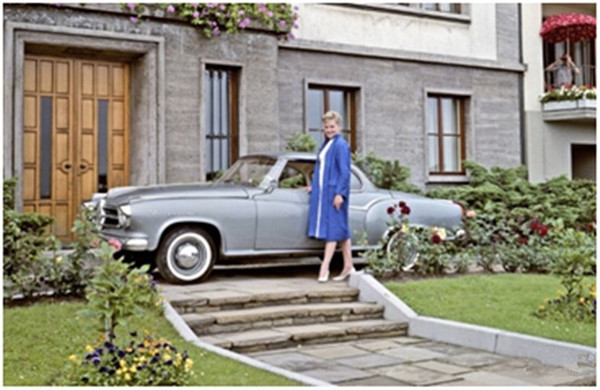关于Isabella 如何得名，有很多传说。其中最具戏剧性的是卡尔·宝沃亲自带他的秘书观看了样车，随口询问道：“丽塔，你来说说，这辆汽车应该叫什么名字？”“这是一辆Isabella！”丽塔本能地脱口而出，谁知卡尔·宝沃竟采纳了这个灵光乍现、未经推敲的名字，而最终这个名字响彻世界。不得不说，BORGWARD（宝沃）公司在汽车命名领域具有在同时代超越对手的审美。在诸如奔驰、欧宝以字母和数字命名车型时，宝沃早已开始用灵动鲜活、易使人产生联想的人名为品牌注入了源源不断的生命力。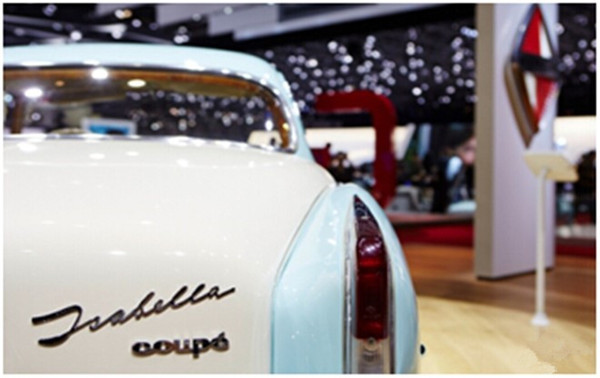“天下没有偶然，偶然不过是化了妆的、戴了面具的必然。”——钱钟书的一席话不知打破了多少侥幸者的清梦。有些人相信冥冥之中有神明在主宰命运，因为那些成败的节点大多来自于偶然机遇的青睐、选择的岔口、突降的灾祸与幸运，它们仿佛具有太多的偶然性，心跳的一瞬命运便随之改变。人们在其前后的境遇截然不同，因此它常常被冠以“决定因素”的谬名。我们可以说偶然间一根雪茄燃起了卡尔·宝沃的汽车生涯，可以说一次偶然一次老友间的推杯换盏推动了直升机的研发，甚至可以说一个秘书的无心之作成就了卡尔·宝沃汽车帝国的巅峰。但事实上，卡尔·宝沃的人生，并非偶然选择了他，而是他拥有了被偶然选中的品质。可正如质变的飞跃需要量变的长久积累，偶然的背后也并非空无一物。卡尔·宝沃如何抓住机会，如何做出选择，如何应对突降的否泰，并非命运之棋无常的落子，而是在他强大的经验累积和随性大胆的性格品质面前，偶然变成了必然。

|分享

•已阅！握爪
•我手滑为你点赞
•128个赞！
•不明觉厉
•阅后既醉
•有钱！任性
•照片太美，我不敢看
•干货！杠杠的
•高大上
•膜拜中！
•请接受我的膝盖
•猫哥/猫妹么么哒
•神吐槽
•我只笑笑不说话
•我想静静
•窒息
##### 热度排行• 阿斯顿·马丁
• 奥迪
• 阿尔法罗密欧
• ALPINA
• 爱驰
• AC Schnitzer
• AITO
• ARCFOX极狐
• AUXUN傲旋

• 巴博斯
• 宝骏
• 宝马
• 保时捷
• 北汽制造
• 奔驰
• 奔腾
• 本田
• 比亚迪
• 标致
• 别克
• 宾利
• 布加迪
• 北汽威旺
• 北京
• 北汽绅宝
• 北汽幻速
• 北汽新能源
• 宝沃
• 比速汽车
• 北汽道达
• 比德文汽车
• 铂驰
• 北汽昌河
• 百智新能源
• 宝骐汽车
• 比克汽车
• 博速

• 昌河
• 长安
• 长城
• 长安商用
• 成功汽车
• 长江EV
• 长安轻车型
• 长安欧尚
• 长安凯程
• 车驰汽车
• 橙仕
• 创维汽车

• 大众
• 道奇
• 东风
• 东风风神
• 东风小康
• 东南
• DS
• 东风风行
• 东风风度
• 东风风光
• 电咖
• 大乘汽车
• 大运
• 东风富康
• 东风·瑞泰特
• 东风新能源
• 东风御风

• 法拉利
• 菲亚特
• 丰田
• 福迪
• 福特
• 福田汽车
• 福汽启腾
• 风诺
• 飞碟汽车
• 飞凡汽车

• GMC
• 广汽传祺
• 广汽吉奥
• 观致
• 国金汽车
• 国机智骏
• 高合汽车
• 广汽埃安

• 哈飞
• 海格
• 海马
• 华泰
• 黄海
• 恒天
• 红旗
• 哈弗
• 华颂
• 华凯
• 华泰新能源
• 汉腾汽车
• 华利
• 红星汽车
• HYCAN合创
• 汉龙汽车
• 恒润汽车
• 宏远汽车
• 华晨新日
• 华骐
• 华梓汽车

• Icona
• IMSA英飒

• Jeep
• 江淮
• 江铃
• 捷豹
• 金杯
• 九龙
• 吉利汽车
• 金旅
• 金龙
• 江铃集团轻汽
• 江铃集团新能源
• 君马汽车
• 捷途
• 钧天
• 捷达
• 几何汽车
• 极氪
• 捷尼赛思
• 金冠汽车

• 开瑞
• 凯迪拉克
• 科尼赛克
• 克莱斯勒
• KTM
• 卡威
• 凯翼
• 康迪
• 康迪电动汽车集团
• 开沃汽车
• 卡升
• 克蒂汽车
• 克慕勒

• 猎豹汽车
• 兰博基尼
• 劳斯莱斯
• 雷克萨斯
• 雷诺
• 理念
• 力帆
• 莲花汽车
• 林肯
• 铃木
• 陆风
• 路虎
• 路特斯
• 领志
• 领克
• 零跑汽车
• Lorinser
• LOCAL MOTORS
• 领途汽车
• 理想汽车
• 罗夫哈特
• 力帆汽车
• LEVC
• LIMGENE凌际
• LUMMA
• 莱茵汽车
• 岚图汽车
• 凌宝汽车
• 龙程汽车

• MG
• MINI
• 马自达
• 玛莎拉蒂
• 迈凯伦
• 摩根
• 迈莎锐
• 迈迈
• 摩登汽车

• 纳智捷
• 哪吒汽车

• 讴歌
• 欧宝
• 欧朗
• 欧拉
• 欧尚汽车

• 帕加尼
• Polestar极星
• 朋克汽车

• 奇瑞
• 启辰
• 起亚
• 前途
• 庆铃汽车
• 骐铃汽车
• 乔治·巴顿

• 日产
• 荣威
• 瑞麒汽车
• 如虎
• 瑞驰
• 瑞驰新能源
• 容大智造
• RAM
• 睿蓝汽车

### S

• 上汽大通
• smart
• 三菱
• 双环
• 双龙
• 斯巴鲁
• 斯柯达
• 萨博
• 思铭
• 赛麟
• SWM斯威汽车
• SRM鑫源
• 思皓
• SHELBY
• 上喆
• SERES赛力斯
• SONGSAN MOTORS
• 速达
• 申龙客车
• 神州

• TESLA
• 泰卡特
• 腾势
• 特斯拉
• 天际汽车
• TECHART
• 坦克

• 威麟
• 威兹曼
• 沃尔沃
• 五菱汽车
• 五十铃
• 潍柴英致
• WEY
• 蔚来
• 威马汽车
• WALD
• 瓦滋
• 伟昊汽车
• 魏牌

• 现代
• 雪佛兰
• 雪铁龙
• 西雅特
• 新特汽车
• 小鹏汽车
• 新宝骏
• 小虎
• 小猬汽车
• 晓奥汽车

• 野马汽车
• 一汽
• 依维柯
• 英菲尼迪
• 永源
• 驭胜
• 云度
• 宇通客车
• 云雀汽车
• 远程汽车
• 一汽凌河
• 雅升汽车
• 野马新能源
• 银隆新能源
• 御捷
• 裕路

• 中华
• 中兴
• 众泰
• 知豆
• 之诺
• 正道汽车
• 智点汽车
• 智己汽车
• 中国重汽VGV
• A
• B
• C
• D
• E
• F
• G
• H
• I
• J
• K
• L
• M
• N
• O
• P
• Q
• R
• S
• T
• U
• V
• W
• X
• Y
• Z

• 阿斯顿·马丁
• 奥迪
• 阿尔法罗密欧
• ALPINA
• 爱驰
• AC Schnitzer
• AITO
• ARCFOX极狐
• AUXUN傲旋

• 巴博斯
• 宝骏
• 宝马
• 保时捷
• 北汽制造
• 奔驰
• 奔腾
• 本田
• 比亚迪
• 标致
• 别克
• 宾利
• 布加迪
• 北汽威旺
• 北京
• 北汽绅宝
• 北汽幻速
• 北汽新能源
• 宝沃
• 比速汽车
• 北汽道达
• 比德文汽车
• 铂驰
• 北汽昌河
• 百智新能源
• 宝骐汽车
• 比克汽车
• 博速

• 昌河
• 长安
• 长城
• 长安商用
• 成功汽车
• 长江EV
• 长安轻车型
• 长安欧尚
• 长安凯程
• 车驰汽车
• 橙仕
• 创维汽车

• 大众
• 道奇
• 东风
• 东风风神
• 东风小康
• 东南
• DS
• 东风风行
• 东风风度
• 东风风光
• 电咖
• 大乘汽车
• 大运
• 东风富康
• 东风·瑞泰特
• 东风新能源
• 东风御风

• 法拉利
• 菲亚特
• 丰田
• 福迪
• 福特
• 福田汽车
• 福汽启腾
• 风诺
• 飞碟汽车
• 飞凡汽车

• GMC
• 广汽传祺
• 广汽吉奥
• 观致
• 国金汽车
• 国机智骏
• 高合汽车
• 广汽埃安

• 哈飞
• 海格
• 海马
• 华泰
• 黄海
• 恒天
• 红旗
• 哈弗
• 华颂
• 华凯
• 华泰新能源
• 汉腾汽车
• 华利
• 红星汽车
• HYCAN合创
• 汉龙汽车
• 恒润汽车
• 宏远汽车
• 华晨新日
• 华骐
• 华梓汽车

• Icona
• IMSA英飒

• Jeep
• 江淮
• 江铃
• 捷豹
• 金杯
• 九龙
• 吉利汽车
• 金旅
• 金龙
• 江铃集团轻汽
• 江铃集团新能源
• 君马汽车
• 捷途
• 钧天
• 捷达
• 几何汽车
• 极氪
• 捷尼赛思
• 金冠汽车

• 开瑞
• 凯迪拉克
• 科尼赛克
• 克莱斯勒
• KTM
• 卡威
• 凯翼
• 康迪
• 康迪电动汽车集团
• 开沃汽车
• 卡升
• 克蒂汽车
• 克慕勒

• 猎豹汽车
• 兰博基尼
• 劳斯莱斯
• 雷克萨斯
• 雷诺
• 理念
• 力帆
• 莲花汽车
• 林肯
• 铃木
• 陆风
• 路虎
• 路特斯
• 领志
• 领克
• 零跑汽车
• Lorinser
• LOCAL MOTORS
• 领途汽车
• 理想汽车
• 罗夫哈特
• 力帆汽车
• LEVC
• LIMGENE凌际
• LUMMA
• 莱茵汽车
• 岚图汽车
• 凌宝汽车
• 龙程汽车

• MG
• MINI
• 马自达
• 玛莎拉蒂
• 迈凯伦
• 摩根
• 迈莎锐
• 迈迈
• 摩登汽车

• 纳智捷
• 哪吒汽车

• 讴歌
• 欧宝
• 欧朗
• 欧拉
• 欧尚汽车

• 帕加尼
• Polestar极星
• 朋克汽车

• 奇瑞
• 启辰
• 起亚
• 前途
• 庆铃汽车
• 骐铃汽车
• 乔治·巴顿

• 日产
• 荣威
• 瑞麒汽车
• 如虎
• 瑞驰
• 瑞驰新能源
• 容大智造
• RAM
• 睿蓝汽车

### S

• 上汽大通
• smart
• 三菱
• 双环
• 双龙
• 斯巴鲁
• 斯柯达
• 萨博
• 思铭
• 赛麟
• SWM斯威汽车
• SRM鑫源
• 思皓
• SHELBY
• 上喆
• SERES赛力斯
• SONGSAN MOTORS
• 速达
• 申龙客车
• 神州

• TESLA
• 泰卡特
• 腾势
• 特斯拉
• 天际汽车
• TECHART
• 坦克

• 威麟
• 威兹曼
• 沃尔沃
• 五菱汽车
• 五十铃
• 潍柴英致
• WEY
• 蔚来
• 威马汽车
• WALD
• 瓦滋
• 伟昊汽车
• 魏牌

• 现代
• 雪佛兰
• 雪铁龙
• 西雅特
• 新特汽车
• 小鹏汽车
• 新宝骏
• 小虎
• 小猬汽车
• 晓奥汽车

• 野马汽车
• 一汽
• 依维柯
• 英菲尼迪
• 永源
• 驭胜
• 云度
• 宇通客车
• 云雀汽车
• 远程汽车
• 一汽凌河
• 雅升汽车
• 野马新能源
• 银隆新能源
• 御捷
• 裕路

• 中华
• 中兴
• 众泰
• 知豆
• 之诺
• 正道汽车
• 智点汽车
• 智己汽车
• 中国重汽VGV
• A
• B
• C
• D
• E
• F
• G
• H
• I
• J
• K
• L
• M
• N
• O
• P
• Q
• R
• S
• T
• U
• V
• W
• X
• Y
• Z

• 阿斯顿·马丁
• 奥迪
• 阿尔法罗密欧
• ALPINA
• 爱驰
• AC Schnitzer
• AITO
• ARCFOX极狐
• AUXUN傲旋

• 巴博斯
• 宝骏
• 宝马
• 保时捷
• 北汽制造
• 奔驰
• 奔腾
• 本田
• 比亚迪
• 标致
• 别克
• 宾利
• 布加迪
• 北汽威旺
• 北京
• 北汽绅宝
• 北汽幻速
• 北汽新能源
• 宝沃
• 比速汽车
• 北汽道达
• 比德文汽车
• 铂驰
• 北汽昌河
• 百智新能源
• 宝骐汽车
• 比克汽车
• 博速

• 昌河
• 长安
• 长城
• 长安商用
• 成功汽车
• 长江EV
• 长安轻车型
• 长安欧尚
• 长安凯程
• 车驰汽车
• 橙仕
• 创维汽车

• 大众
• 道奇
• 东风
• 东风风神
• 东风小康
• 东南
• DS
• 东风风行
• 东风风度
• 东风风光
• 电咖
• 大乘汽车
• 大运
• 东风富康
• 东风·瑞泰特
• 东风新能源
• 东风御风

• 法拉利
• 菲亚特
• 丰田
• 福迪
• 福特
• 福田汽车
• 福汽启腾
• 风诺
• 飞碟汽车
• 飞凡汽车

• GMC
• 广汽传祺
• 广汽吉奥
• 观致
• 国金汽车
• 国机智骏
• 高合汽车
• 广汽埃安

• 哈飞
• 海格
• 海马
• 华泰
• 黄海
• 恒天
• 红旗
• 哈弗
• 华颂
• 华凯
• 华泰新能源
• 汉腾汽车
• 华利
• 红星汽车
• HYCAN合创
• 汉龙汽车
• 恒润汽车
• 宏远汽车
• 华晨新日
• 华骐
• 华梓汽车

• Icona
• IMSA英飒

• Jeep
• 江淮
• 江铃
• 捷豹
• 金杯
• 九龙
• 吉利汽车
• 金旅
• 金龙
• 江铃集团轻汽
• 江铃集团新能源
• 君马汽车
• 捷途
• 钧天
• 捷达
• 几何汽车
• 极氪
• 捷尼赛思
• 金冠汽车

• 开瑞
• 凯迪拉克
• 科尼赛克
• 克莱斯勒
• KTM
• 卡威
• 凯翼
• 康迪
• 康迪电动汽车集团
• 开沃汽车
• 卡升
• 克蒂汽车
• 克慕勒

• 猎豹汽车
• 兰博基尼
• 劳斯莱斯
• 雷克萨斯
• 雷诺
• 理念
• 力帆
• 莲花汽车
• 林肯
• 铃木
• 陆风
• 路虎
• 路特斯
• 领志
• 领克
• 零跑汽车
• Lorinser
• LOCAL MOTORS
• 领途汽车
• 理想汽车
• 罗夫哈特
• 力帆汽车
• LEVC
• LIMGENE凌际
• LUMMA
• 莱茵汽车
• 岚图汽车
• 凌宝汽车
• 龙程汽车

• MG
• MINI
• 马自达
• 玛莎拉蒂
• 迈凯伦
• 摩根
• 迈莎锐
• 迈迈
• 摩登汽车

• 纳智捷
• 哪吒汽车

• 讴歌
• 欧宝
• 欧朗
• 欧拉
• 欧尚汽车

• 帕加尼
• Polestar极星
• 朋克汽车

• 奇瑞
• 启辰
• 起亚
• 前途
• 庆铃汽车
• 骐铃汽车
• 乔治·巴顿

• 日产
• 荣威
• 瑞麒汽车
• 如虎
• 瑞驰
• 瑞驰新能源
• 容大智造
• RAM
• 睿蓝汽车

### S

• 上汽大通
• smart
• 三菱
• 双环
• 双龙
• 斯巴鲁
• 斯柯达
• 萨博
• 思铭
• 赛麟
• SWM斯威汽车
• SRM鑫源
• 思皓
• SHELBY
• 上喆
• SERES赛力斯
• SONGSAN MOTORS
• 速达
• 申龙客车
• 神州

• TESLA
• 泰卡特
• 腾势
• 特斯拉
• 天际汽车
• TECHART
• 坦克

• 威麟
• 威兹曼
• 沃尔沃
• 五菱汽车
• 五十铃
• 潍柴英致
• WEY
• 蔚来
• 威马汽车
• WALD
• 瓦滋
• 伟昊汽车
• 魏牌

• 现代
• 雪佛兰
• 雪铁龙
• 西雅特
• 新特汽车
• 小鹏汽车
• 新宝骏
• 小虎
• 小猬汽车
• 晓奥汽车

• 野马汽车
• 一汽
• 依维柯
• 英菲尼迪
• 永源
• 驭胜
• 云度
• 宇通客车
• 云雀汽车
• 远程汽车
• 一汽凌河
• 雅升汽车
• 野马新能源
• 银隆新能源
• 御捷
• 裕路

• 中华
• 中兴
• 众泰
• 知豆
• 之诺
• 正道汽车
• 智点汽车
• 智己汽车
• 中国重汽VGV
• A
• B
• C
• D
• E
• F
• G
• H
• I
• J
• K
• L
• M
• N
• O
• P
• Q
• R
• S
• T
• U
• V
• W
• X
• Y
• Z